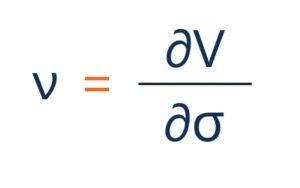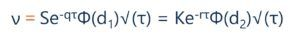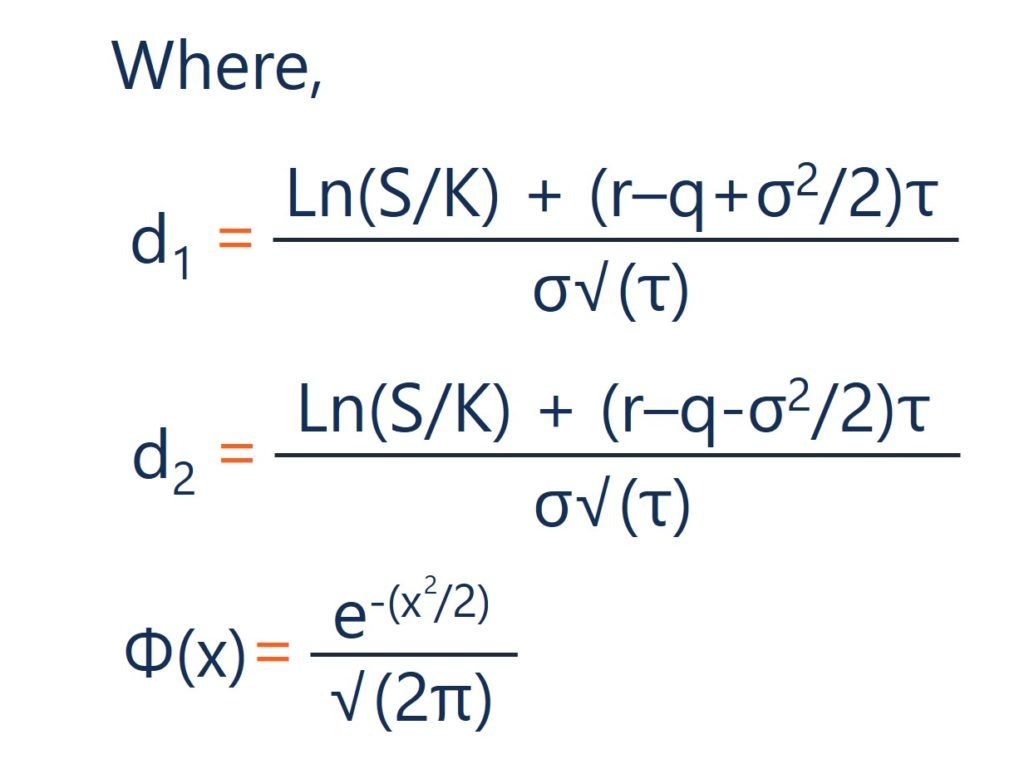# Vega (ν)

The sensitivity of the option price to a 1% change in volatility

## What is Vega?

Vega falls under the series of sensitivity measures called the Greeks. Vega is not a Greek letter; however, it is denoted by the Greek letter nu (ν). The Greeks are measures used to assess derivatives and are often referred to as risk measures, hedge parameters, or risk sensitivities. Vega measures an option’s sensitivity to the underlying asset’s volatility. It is very important in option pricing and is expressed as the change in the value of the option as volatility changes by a 1% increment.### Quick Summary of Points

• Vega measures the sensitivity of the option price to a 1% change in the implied volatility
• Implied volatility refers to the expected volatility of the underlying asset
• Higher volatility generally means a higher extrinsic value priced into the premium of an option
• Vega can be used to assess the potential of an option to increase in value before the expiration date

### What is Implied Volatility?

Vega can be thought of as the change in the value of a derivative, to a 1% change in the implied volatility of the underlying asset. To understand what this means, we must first understand what implied volatility is, and how it is measured.

Implied volatility refers to the expected volatility of the underlying asset. Implied volatility can be shortened to IV or just volatility. A higher IV means there is more uncertainty around the price of the stock. As IV increases, you would expect to see larger swings in the price.

IV is expressed as a percentage change associated with one standard deviation, annualized. An implied volatility of 20% would mean that the standard deviation over the next year would be a 20% change in price. In a normal distribution, it would be a 68.2% probability of a 20% change in price. If the price of the underlying asset is \$100, then you would expect the stock to be between \$80 and \$120 in the next year.

### How to Interpret Vega?

Vega is generally positive for both call options and put options that have time until the expiration date. Vega measures the sensitivity of the option price to a 1% change in the implied volatility. The units of vega are \$/σ; however, like the other Greeks, the units are often left out. An option with a vega of 0.10 would mean that for every 1% change in the IV, the option price should change by \$0.10.

There are three main things that affect vega. It is affected by the time until expiration, the strike price relative to the underlying asset’s spot price, and the implied volatility. The longer time there is until the expiration of an option, the higher the extrinsic value of the premium. The reason the extrinsic value is the ability to hold the option and the opportunity for the option to gain value as the underlying asset moves in price.

Higher volatility generally means a higher extrinsic value priced into the premium of an option. The reason for this is that the time value is heavily influenced by the implied volatility. A higher IV means a greater chance for the underlying asset to move in price and the option to increase in value before the expiration date.

The option strike price relative to the asset spot price is important too. If an option is very out of the money, the vega tends to be smaller. It is because even if volatility changes, there is still not a very high chance that the option will end up in the money, meaning the price will not show a significant difference.

Let us look at a hypothetical call option with a premium of \$5 and an underlying asset with a price of \$100. If the IV is 20% and the vega of the option is 0.10, what would happen to the option price if the IV rose to 22%? The 2% increase should mean that the change in price would be an increase of 2 x 0.10 = \$0.20. You would expect the price to increase from \$5.00 to \$5.20. If the IV instead fell by 2%, you would expect a decrease in the price of \$0.20, resulting in a price of \$4.80.

### What is Vega Used For In Options

Vega can be used in determining the time value of an option. The extrinsic value is very important in understanding option pricing and can be used to assess the potential of an option to increase in value before the expiration date. An option’s vega will generally not be a static number. As an option’s implied volatility rises or falls, and it moves closer to expiration, the vega changes and traders often monitor vega to assess how an options price might move.

Traders often refer to being long or short vega. Being long vega means that they are holding a long position and will benefit from a rise in implied volatility. Being short vega means the trader holds a short position and will benefit if the implied volatility falls.

### How is Vega Calculated?

The general form of vega can be represented by:Where:

• ∂ – the first derivative
• V – the option’s price (theoretical value)
• σ – the volatility of the underlying asset

Under the Black-Scholes model, the calculation for vega is given by:Where:

• S – the stock price
• K – the strike price
• r – the risk-free rate
• q – the annual dividend yield
• τ – time until expiration
• σ – the volatility